# HSSlive: Plus One & Plus Two Notes & Solutions for Kerala State Board

## BSEB Class 10 Maths Chapter 12 Areas Related to Circles Ex 12.3 Textbook Solutions PDF: Download Bihar Board STD 10th Maths Chapter 12 Areas Related to Circles Ex 12.3 Book AnswersBSEB Class 10 Maths Chapter 12 Areas Related to Circles Ex 12.3 Textbook Solutions PDF: Download Bihar Board STD 10th Maths Chapter 12 Areas Related to Circles Ex 12.3 Book Answers

BSEB Class 10th Maths Chapter 12 Areas Related to Circles Ex 12.3 Textbooks Solutions and answers for students are now available in pdf format. Bihar Board Class 10th Maths Chapter 12 Areas Related to Circles Ex 12.3 Book answers and solutions are one of the most important study materials for any student. The Bihar Board Class 10th Maths Chapter 12 Areas Related to Circles Ex 12.3 books are published by the Bihar Board Publishers. These Bihar Board Class 10th Maths Chapter 12 Areas Related to Circles Ex 12.3 textbooks are prepared by a group of expert faculty members. Students can download these BSEB STD 10th Maths Chapter 12 Areas Related to Circles Ex 12.3 book solutions pdf online from this page.

## Bihar Board Class 10th Maths Chapter 12 Areas Related to Circles Ex 12.3 Books Solutions

 Board BSEB Materials Textbook Solutions/Guide Format DOC/PDF Class 10th Subject Maths Chapter 12 Areas Related to Circles Ex 12.3 Chapters All Provider Hsslive

## How to download Bihar Board Class 10th Maths Chapter 12 Areas Related to Circles Ex 12.3 Textbook Solutions Answers PDF Online?

2. Click on the Bihar Board Class 10th Maths Chapter 12 Areas Related to Circles Ex 12.3 Answers.
3. Look for your Bihar Board STD 10th Maths Chapter 12 Areas Related to Circles Ex 12.3 Textbooks PDF.
4. Now download or read the Bihar Board Class 10th Maths Chapter 12 Areas Related to Circles Ex 12.3 Textbook Solutions for PDF Free.

## BSEB Class 10th Maths Chapter 12 Areas Related to Circles Ex 12.3 Textbooks Solutions with Answer PDF Download

Find below the list of all BSEB Class 10th Maths Chapter 12 Areas Related to Circles Ex 12.3 Textbook Solutions for PDF’s for you to download and prepare for the upcoming exams:

## BSEB Bihar Board Class 10th Maths Solutions Chapter 12 Areas Related to Circles Ex 12.3

Question 1.
Find the area of the shaded region in the figure, if PQ = 24 cm, PR = 7 cm and O is the centre of the circle.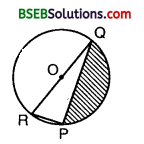[Annual Paper (Delhi) 2009]
Solution:
Since ROQ is a diameter, therefore ∠RPQ = 90°.
RQ² = RP² + PQ²
or RQ² = 7² + 24² = 49 + 576 = 625
or RQ = 625‾‾‾‾√ cm = 25 cm
∴ Radius r = 12 RQ = 252 cm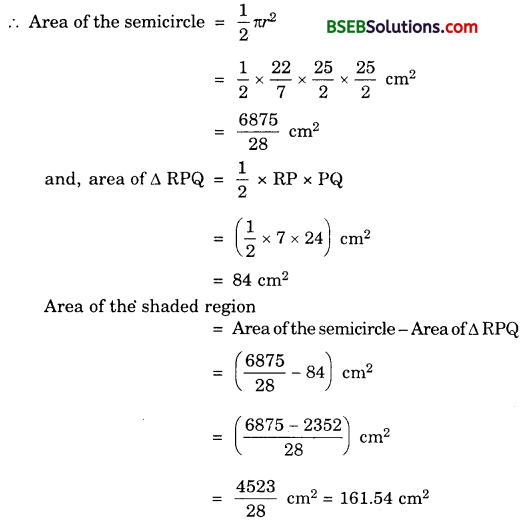Question 2.
Find the area of the shaded region in the figure, if radii of the two concentric circles with centre O are 7 cm and 14 cm respectively and ∠AOC = 40°.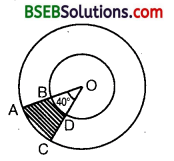Solution:
Area of the shaded region = Area of sector AOC – Area of sector OBD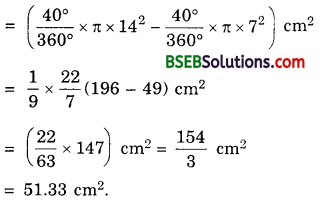Question 3.
Find the area of the shaded A region in the figure, if ABCD is a square of side 14 cm and APD and BPC are semicircles.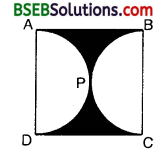Solution:
Area of the square ABCD = (14)² cm² = 196 cm²
Diameter of the semicircles = AD or BC = 14 cm
∴ Radius of each semicircle = 142 cm = 7 cm
∴ Area of the both semicircular regions
= 2 x 12πr² = πr²
= (227 x 49) cm² = 154 cm²
∴ Area of the shaded regions = Area of the square ABCD – Area of the both semicircular regions
= (196 – 154) cm² – 42 cm².

Question 4.
Find the area of the shaded region in the figure, where a circular arc of radius 6 cm has been drawn with vertex O of an equilateral triangle OAB of side 12 cm as centre.
Solution:
Area of the circular portion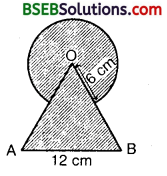Area of the equilateral ∆ OAB
3√4(side)² = (3√4 x 144) cm²
= 363‾√ cm²
Area of the shaded region = Area of circular portion + Area of the equilateral triangle
= (6607+363‾√) cm²

Question 5.
From each corner of a square of side 4 cm a quadrant of a circle of radius 1 cm is cut and also a circle of diameter 2 cm is cut as shown in the figure. Find the area of the remaining portion of the square.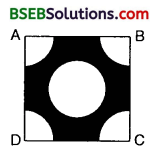Solution:
The area of the square ABCD = (side)²
= 4² cm²
= 16 cm²
The sum of the areas of the four quadrants at the four corners of the square
= The area of a circle of radius 1 cm
= 227 x 1² cm²
= 227 cm²
The area of the circle of diameter 2 cm, i.e., radius 1 cm²
= 227 x 1² cm²
= 227 cm²
Area of the remaining portion of the square = The area of the square ABCD – The sum of the areas of 4 quadrants at the four corners of the square – The area of the circle of diameter 2 cm
= (16 – 227 – 227) cm²
= (112−22−227) cm²
= 687 cm² – 9.71 cm²

Question 6.
In a circular table cover of radius 32 cm, a design is formed leaving an equilateral triangle ABC in the middle as shown in the figure. Find the area of the design (shaded region).Solution:
Let ABC be an equilateral triangle and let 0 be the circumcentre of the circumcircle of radius 32 cm.Draw OM ⊥ BC.
Now, ∠BOM = 12 x 120° = 60°
So, from ∆ BOM, we haveHence, area of ∆ BOC
= 12 BC x OM
= 12 x 323‾√ x 16 cm²
Area of ∆ ABC = 3 x Area of ∆ BOC
= (3 x 12 x 323‾√ x 16) cm²
= 7683‾√ cm²
∴ Area of the design (i.e., shaded region) = Area of the circle – Area of ∆ ABC
= (225287−7683‾√) cm²

Question 7.
In the figure, ABCD is a square of side 14 cm. With centres A, B, C and D, four circles are drawn such that each circle touch externally two of the remaining three circles. Find the area of the shaded region.Solution:
The area of the square ABCD = (side)² = 142 cm²
= 196 cm².
The sum of the areas of the four quadrants at the four corners of the square
= The area of a circle of radius 142 cm = 7 cm²
= π(7)² cm² =(227 x 49) cm² = 154 cm²
Area of the shaded region = The area of the square ABCD – The sum of the areas of four quadrants at the four corners of the square
= (196 – 154) cm² = 42 cm²

Question 8.
Figure depicts a racing track whose left and right ends are semicircular.The distance between the two inner parallel line segments is 60 m and they are each 106 m long. If the track is 10 m wide, find
(i) the distance around the track along its inner edge.
(ii) the area of the track.
Solution:
We have OB = O’C = 30 m
and, AB = CD = 10 m
OA = O’D = (30 + 10) m = 40 m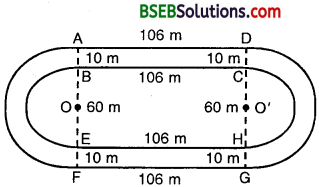(i) the distance around the track along its inner edge.
= BC + EH + 2 x circumference of the semicircle of radius OB = 30 m
= ( 106 + 106 + 2 x 12 x 2π(30) ) m
= ( 212 + 2 x 227 x 3o) m
= ( 212 + 13207 )m = (1484+13207) m
= 28047 m
m = 400.57 m

(ii) Area of the track = Area of the shaded region = Area of rectangle ABCD + Area of rectangle EFGH + 2(Area of the semicircle of radius 40 m – Area of the semicircle with radius 30 m)Question 9.
In the figure, AB and CD are two diameters of a circle (with centre O) perpendicular to each other and OD is the diameter of the smaller circle. If OA = 7 cm, find the area of the shaded region.Solution:
Area of the sector OCB = (90∘360∘×227×7×7) cm²
= 772 cm²
Area of ∆ OCB = 12 x OC x OB
= 12 x 7 x 7 cm²
= 492 cm²
The area of the segment BPC = Area of the sector OCB – Area of the A OCB
= (772 – 492) cm²
= 282 cm²
= 14 cm²
Similarly, the area of the segment AQC
= 14 cm²
Also,the area of the circle with DO as diameter
= (227 x 72 x 72) cm² = 772 cm²
Hence,the total area of the shaded region
= (14 + 14 + 772) cm²
= (28+28+772) cm²
= 1332 cm²
= 66.5 cm²

Question 10.
The area of an equilateral triangle ABC is 17320.5 cm². With each vertex of the triangle as centre, a circle is drawn with radius equal to half the length of the side of the triangle (see figure). Find the area of the shaded region.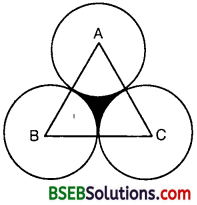(Use π = 3.14 and 3‾√ = 1.73205)
Solution:
Let each side of the triangle be a cm. Then,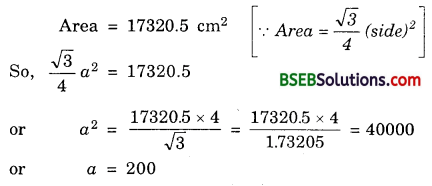Thus, radius of each circle is 2002 cm = 100 cm.
= Area of ∆ ABC – 3 x (Area of a sector of angle 60° in a circle of radius 100 cm)
= [17320.5 – 3(60∘360∘ x 3.14 x 100 x 100)] cm²
= (17320.5 – 15700) cm² = 1620.5 cm²

Question 11.
On a square handkerchief, nine circular designs each of radius 7 cm are made (see figure). Find the area of the remaining portion of the handkerchief.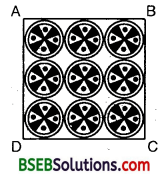Solution:
Side of the square ABCD
= AB – 3 x diameter of circular design
= 3 x (2 x 7) cm – 42 cm
∴ Area of the square ABCD
= (42 x 42) cm² = 1764 cm²
Area of one circular Resign
= πr² = (227 x 7 x 7) cm²
= 154 cm²
∴ Area of 9 such designs
= (9 x 154) cm² = 1386 cm²
∴ Area of the remaining portion of the handkerchief
= Area of the square ABCD – Area of 9 circular designs
= (1764 – 1386) cm² – 378 cm²

Question 12.
In the figure, OACB is a quadrant of a circle with centre O and radius 3.5 cm. If OD = 2 cm, find the area of theSolution:Hence, area of the shaded region = Area of quadrant – Area of ∆ BOD
= (778 – 72) cm² = (77−288) cm²
= 498 cm² = 6.125 cm²

Question 13.
In the figure, a square OABC is inscribed in a quadrant OPBQ. If OA = 20 cm, find the area of the shaded region. (Use π = 3.14)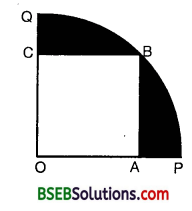Solution:
= 202+202‾‾‾‾‾‾‾‾‾√ cm
= 201+1‾‾‾‾‾√ cm
= 202‾√ cm
= 14πr²
= 14 x 314 x (202‾√)² cm²
= ( 14 x 3.14 x 80o) cm² = 628 cm²
Area of the square OABC = (20) cm² = 400 cm²
Hence, area of the shaded region = Area of quadrant – Area of square OABC
= (628 – 400) cm² = 228 cm².

Question 14.
AB and CD are respectively arcs of two concentric circles of radii 21 cm and 7 cm and centre O (see figure). If ∠AOB = 30°, find the area of the shaded region.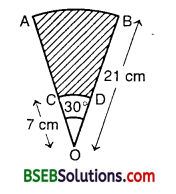Solution:
Let A1 and A2 be the areas of sector OAB and OCD respectively. Then,
A1 = Area of a sector of angle 30° in a circle of radius 21 cmA2 = Area of a sector of angle 30° in a circle of radius 7 cm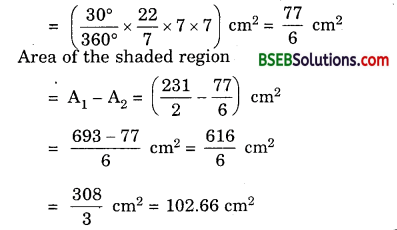Question 15.
In the figure, ABC is a quadrant of a circle of radius 14 cm and a semicircle is drawn with BC as diameter. Find the area of the shaded region.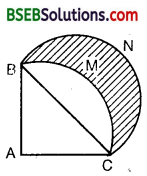[Annual Paper (Foreign) 2008]
Solution:
Here the radius of the quadrant with A as the centre is 14 cm.
Then, the area of the quadrant ABMC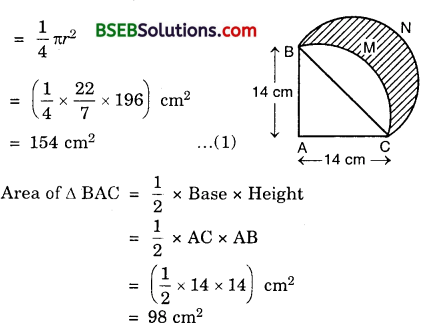Area of the segment BMC of the circle = Area of the quadrant ABMC – Area of ∆ BAC
= (154 – 98) cm²
= 56 cm²
Now, since AC = AB = 14 cm and ∠BAC = 90°, therefore by Pythagoras Theorem,
BC = AC2+AB2‾‾‾‾‾‾‾‾‾‾‾√
= 142+142‾‾‾‾‾‾‾‾‾√
= 142‾√ cm
∴ Radius of the semicircle BNC = 12 x 142‾√ cm = 72‾√ cm
∴ Area of the semicircle BNC
= (72‾√ )² cm²
= (12×227×98) cm² = 154 cm²
Hence, the area of the shaded region
= the area of the region between two arcs BMC and BNC = The area of semicircle BNC – The area of the segment of the circle BMC
= (154 – 56) cm² = 98 cm²

Question 16.
Calculate the area of the designed region in figure common between the two quadrants of circles of radius 8 cm each.Solution:
Here, 8 cm is the radius of each of the quadrants ABMD and BNDC.
Sum of their areas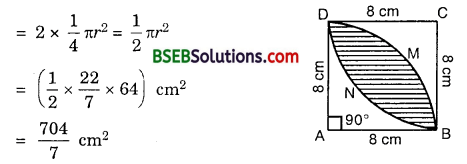Area of the square ABCD = (8 x 8) cm² = 64 cm²
Area of the designed region = Area of the shaded region
= Sum of the areas of quadrants – Area of the square ABCD
= (7047−64) cm² = (704−4487) cm²
= 2567 cm²
= 36.57 cm²

## Bihar Board Class 10th Maths Chapter 12 Areas Related to Circles Ex 12.3 Textbooks for Exam Preparations

Bihar Board Class 10th Maths Chapter 12 Areas Related to Circles Ex 12.3 Textbook Solutions can be of great help in your Bihar Board Class 10th Maths Chapter 12 Areas Related to Circles Ex 12.3 exam preparation. The BSEB STD 10th Maths Chapter 12 Areas Related to Circles Ex 12.3 Textbooks study material, used with the English medium textbooks, can help you complete the entire Class 10th Maths Chapter 12 Areas Related to Circles Ex 12.3 Books State Board syllabus with maximum efficiency.

## FAQs Regarding Bihar Board Class 10th Maths Chapter 12 Areas Related to Circles Ex 12.3 Textbook Solutions

#### How to get BSEB Class 10th Maths Chapter 12 Areas Related to Circles Ex 12.3 Textbook Answers??

Students can download the Bihar Board Class 10 Maths Chapter 12 Areas Related to Circles Ex 12.3 Answers PDF from the links provided above.

#### Can we get a Bihar Board Book PDF for all Classes?

Yes you can get Bihar Board Text Book PDF for all classes using the links provided in the above article.

## Important Terms

Bihar Board Class 10th Maths Chapter 12 Areas Related to Circles Ex 12.3, BSEB Class 10th Maths Chapter 12 Areas Related to Circles Ex 12.3 Textbooks, Bihar Board Class 10th Maths Chapter 12 Areas Related to Circles Ex 12.3, Bihar Board Class 10th Maths Chapter 12 Areas Related to Circles Ex 12.3 Textbook solutions, BSEB Class 10th Maths Chapter 12 Areas Related to Circles Ex 12.3 Textbooks Solutions, Bihar Board STD 10th Maths Chapter 12 Areas Related to Circles Ex 12.3, BSEB STD 10th Maths Chapter 12 Areas Related to Circles Ex 12.3 Textbooks, Bihar Board STD 10th Maths Chapter 12 Areas Related to Circles Ex 12.3, Bihar Board STD 10th Maths Chapter 12 Areas Related to Circles Ex 12.3 Textbook solutions, BSEB STD 10th Maths Chapter 12 Areas Related to Circles Ex 12.3 Textbooks Solutions,
Share: